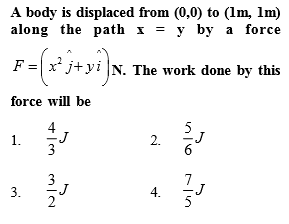You’ve reached the end of your free Videos limit.
#3 | Work Done by Variable Force
(Physics) > Work,Energy and Power
Related Practice Questions :Complete Question Bank + Test Series
Complete Question Bank

Difficulty Level:

A body of mass m is moving in a circle of radius r with a constant speed v. The force on the body is $\frac{m{v}^{2}}{r}$ and is directed towards the centre. What is the work done by this force in moving the body over half the circumference of the circle

(1) $\frac{m{v}^{2}}{r}×\pi r$

(2) Zero

(3) $\frac{m{v}^{2}}{{r}^{2}}$

(4) $\frac{\pi {r}^{2}}{m{v}^{2}}$

Complete Question Bank + Test Series
Complete Question Bank

Difficulty Level:

When a body moves with a constant speed along a circle

(1) No work is done on it

(2) No acceleration is produced in the body

(3) No force acts on the body

(4) Its velocity remains constant

Complete Question Bank + Test Series
Complete Question Bank

Difficulty Level:

A sphere of mass m is tied to end of a string of length l and rotated through the other end along a horizontal circular path with speed v. The work done by centripetal force in full horizontal circle is

(1) 0

(2) $\left(\frac{m{v}^{2}}{l}\right) . 2\pi l$

(3) $\text{mg\hspace{0.17em}.\hspace{0.17em}2πl}$

(4) $\left(\frac{m{v}^{2}}{l}\right) . \left(l\right)$

Complete Question Bank + Test Series
Complete Question Bank

Difficulty Level:

A body of mass 6kg is under a force which causes displacement in it given by $S=\frac{{t}^{2}}{4}$ metres where t is time. The work done by the force in 2 seconds is-

(1) 12 J

(2) 9 J

(3) 6 J

(4) 3 J